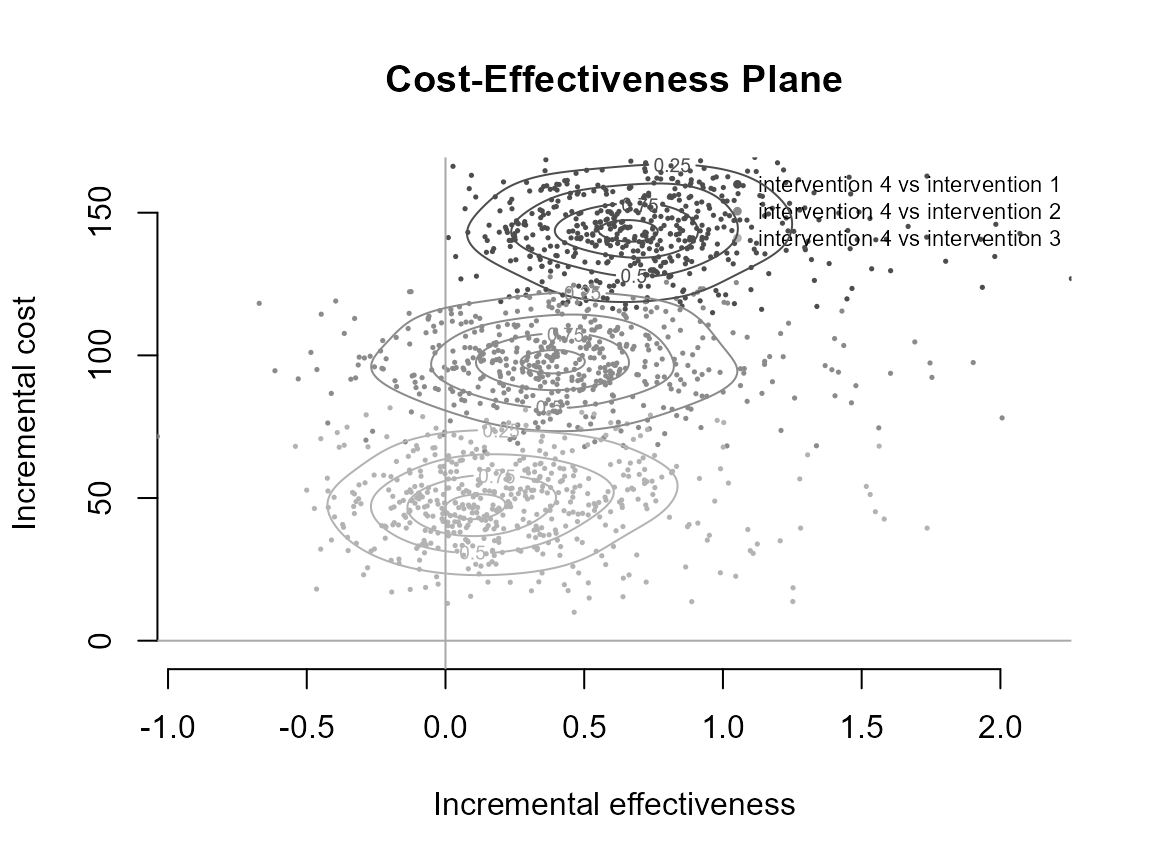## Introduction

The intention of this vignette is to show how to plot different styles of contour plot using the BCEA package and the contour() and contour2() functions.

#### R code

To calculate these in BCEA we use the bcea() function.

data("Vaccine")
he <- bcea(eff, cost, ref = 2)

The plot defaults to base R plotting. Type of plot can be set explicitly using the graph argument.

contour(he, graph = "base")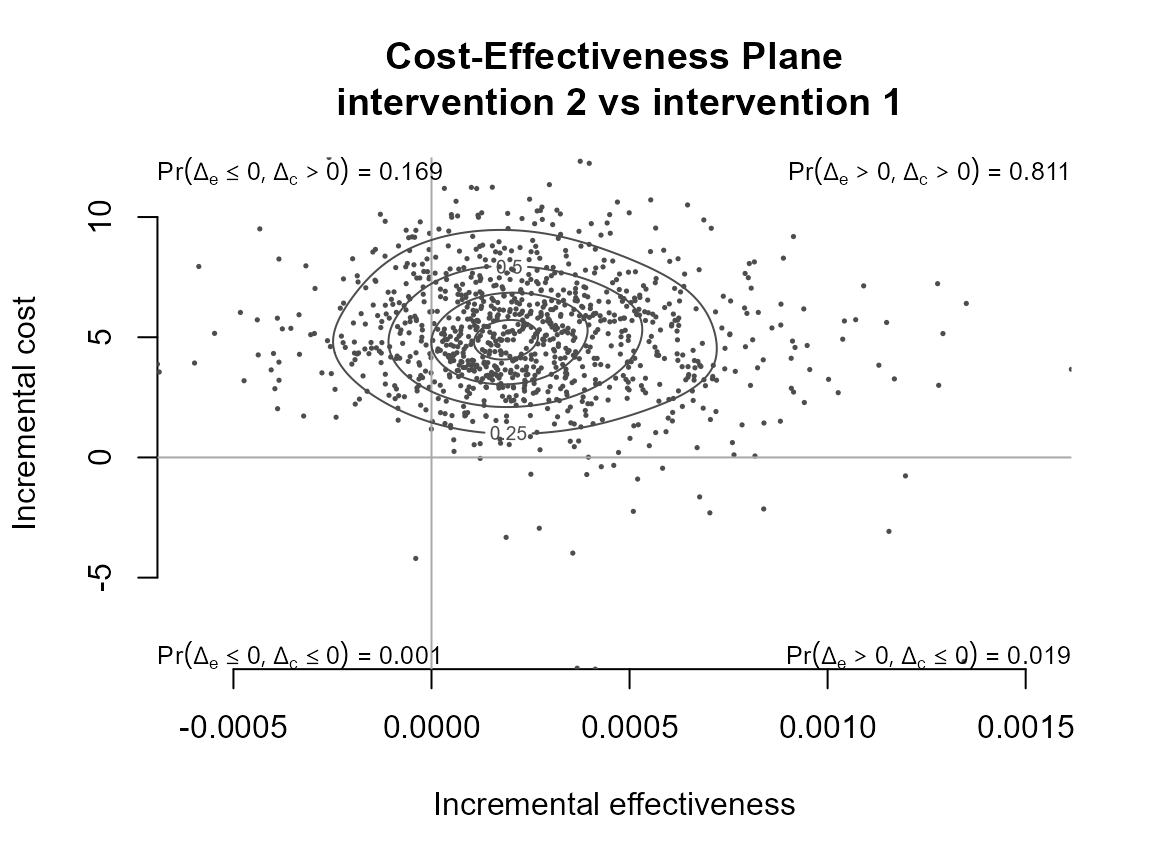contour(he, graph = "ggplot2")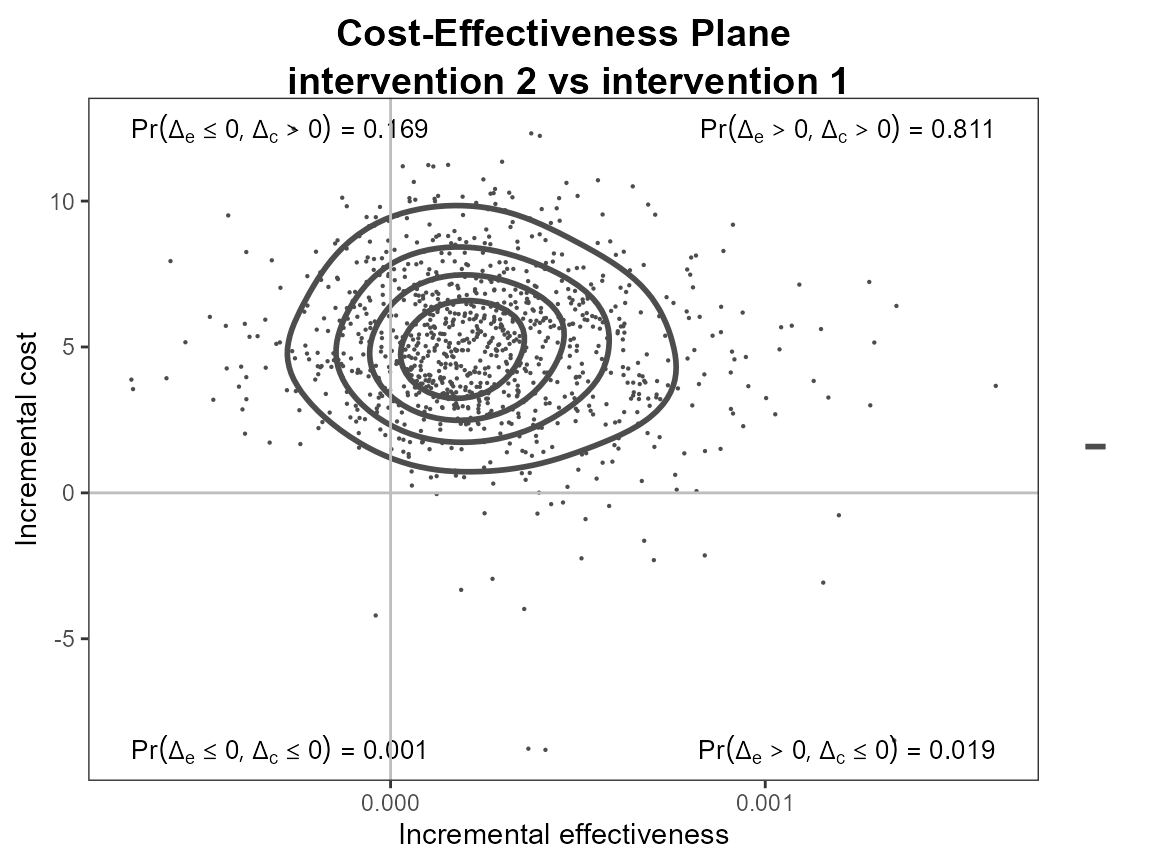# ceac.plot(he, graph = "plotly")

User-defined contour levels can be provided. The levels and nlevels arguments specify the quantiles or number of levels. The base R levels arguments are kept for back-compatibility and the ggplot2 style arguments are used in the associated plot.

contour(he, levels = c(0.2, 0.8))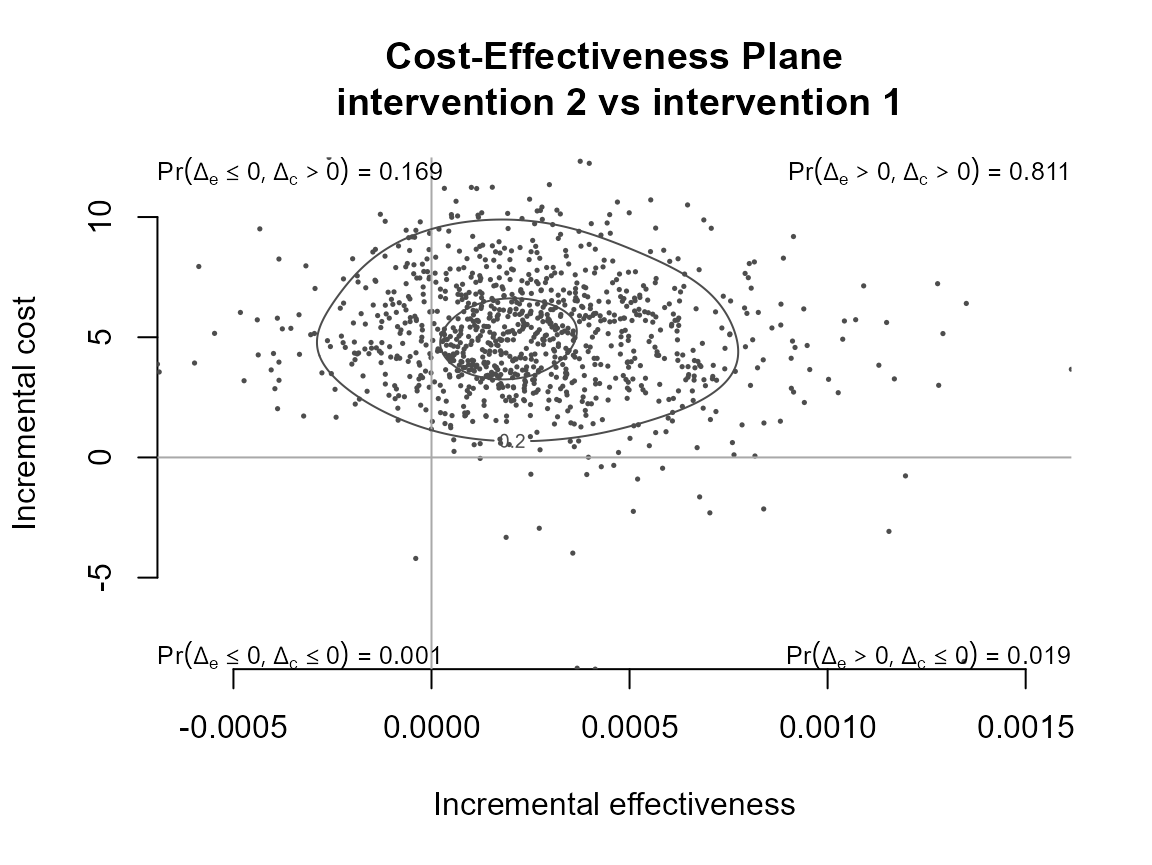contour(he, graph = "ggplot2", contour = list(breaks = c(0.2, 0.8)))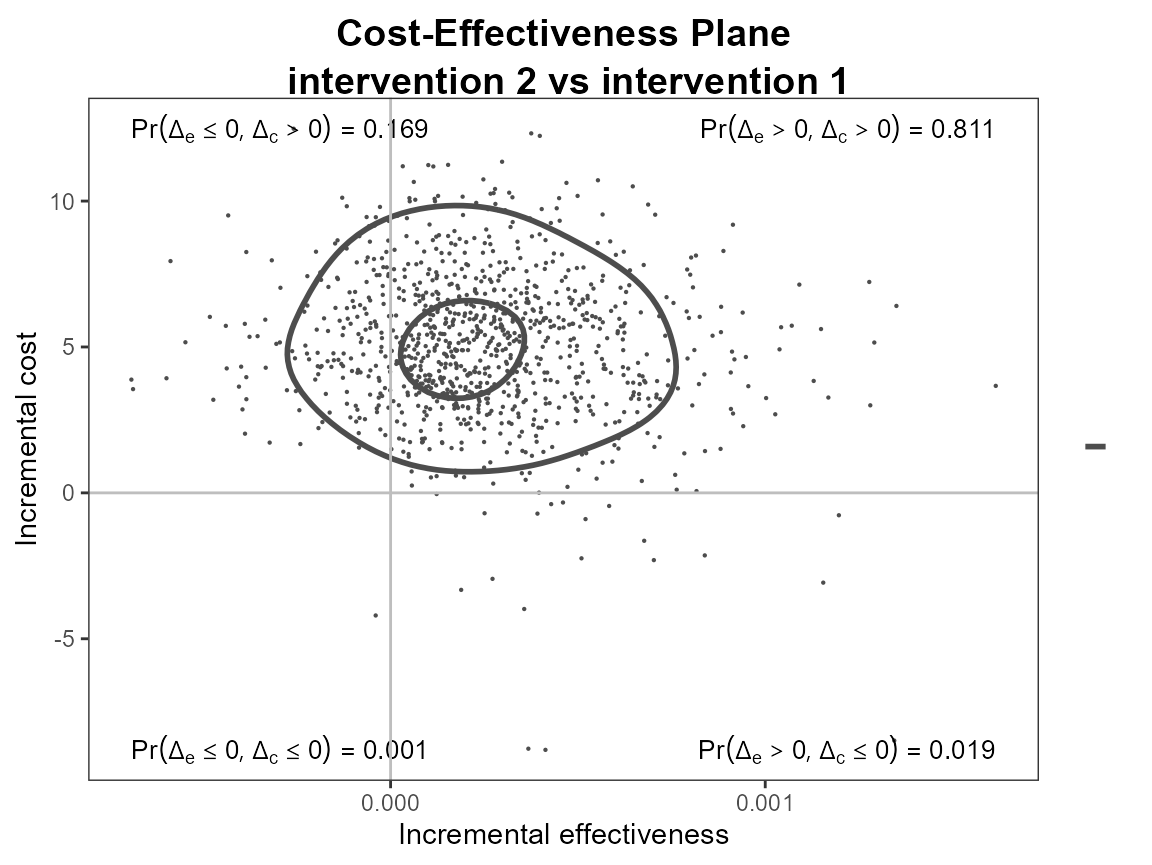Other plotting arguments can be specified such as title, line colour and thickness and type of point.

contour(he,
graph = "ggplot2",
title = "my title",
point = list(color = "blue", shape = 2, size = 5),
contour = list(size = 2))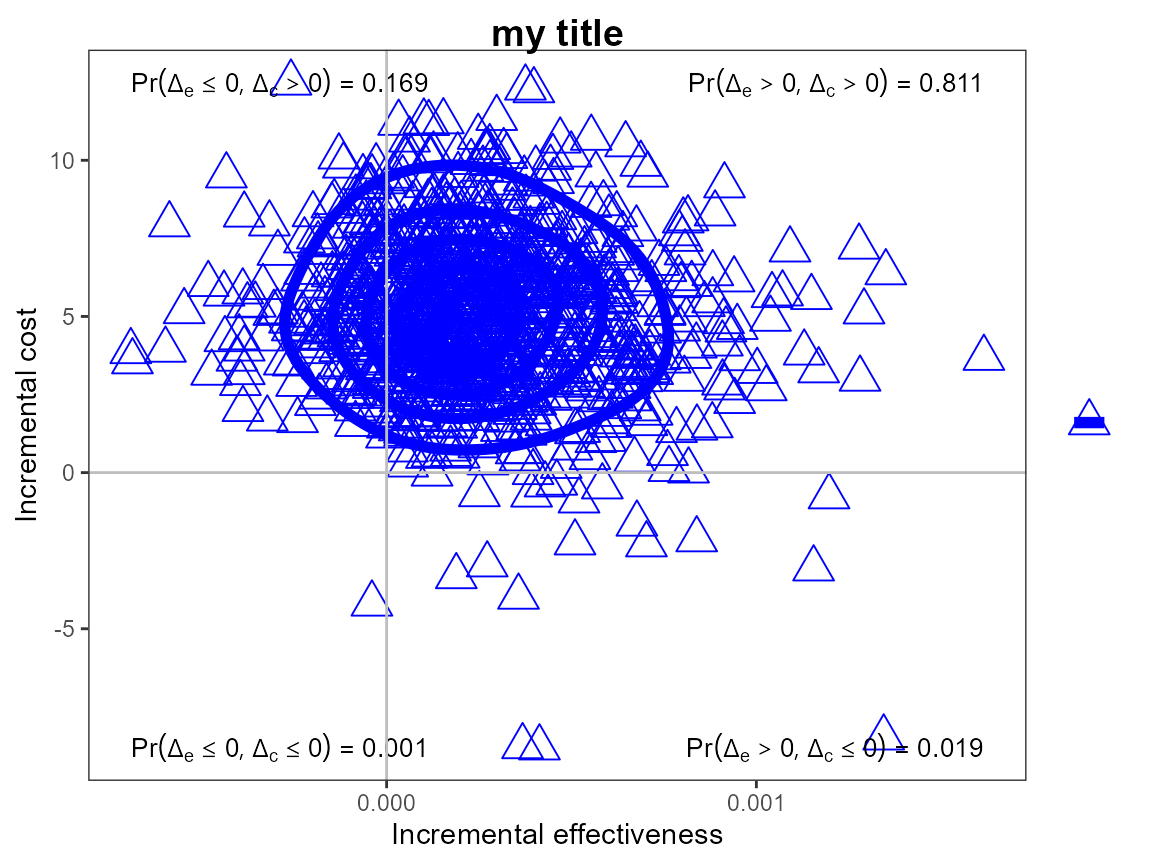contour(he,
graph = "base",
title = "my title",
point = list(color = "blue", shape = 2, size = 2),
contour = list(size = 2))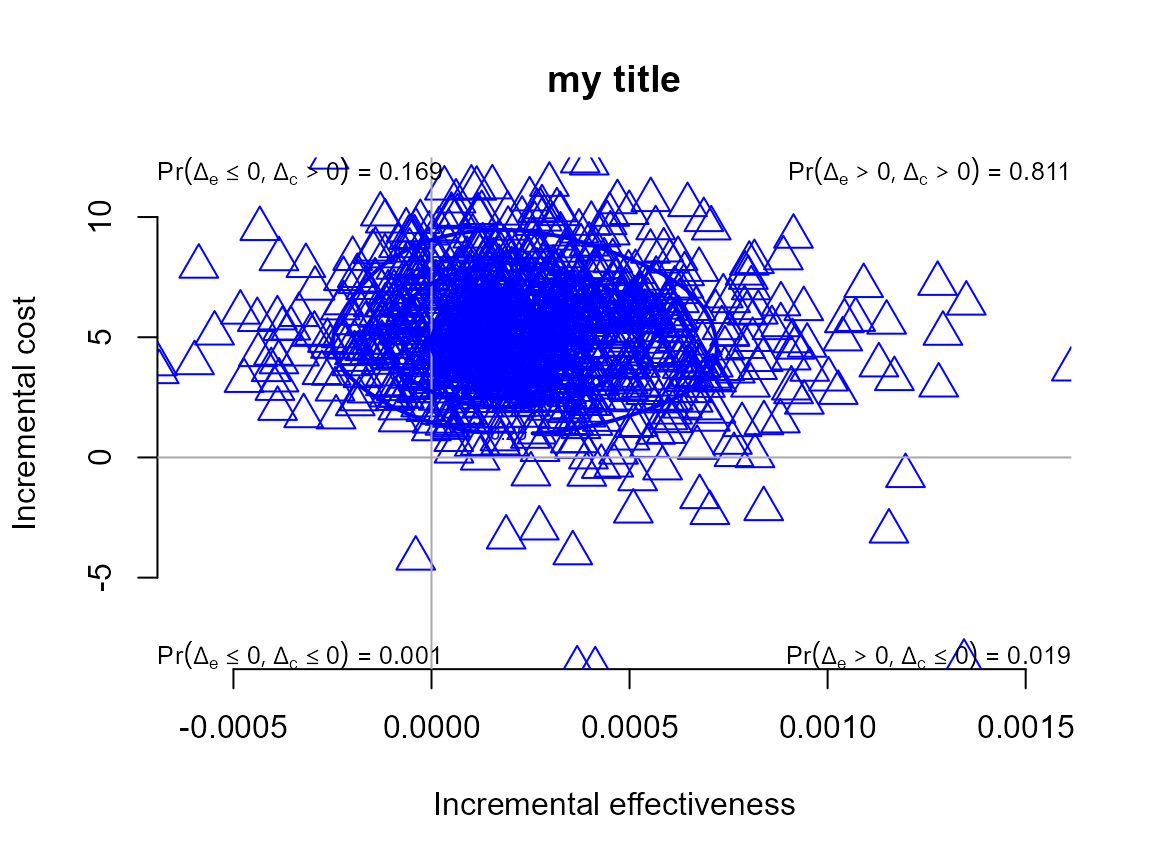Alternatively, the contour2() function is essentially a wrapper for ceplane.plot() with the addition of contour lines.

contour2(he, graph = "base")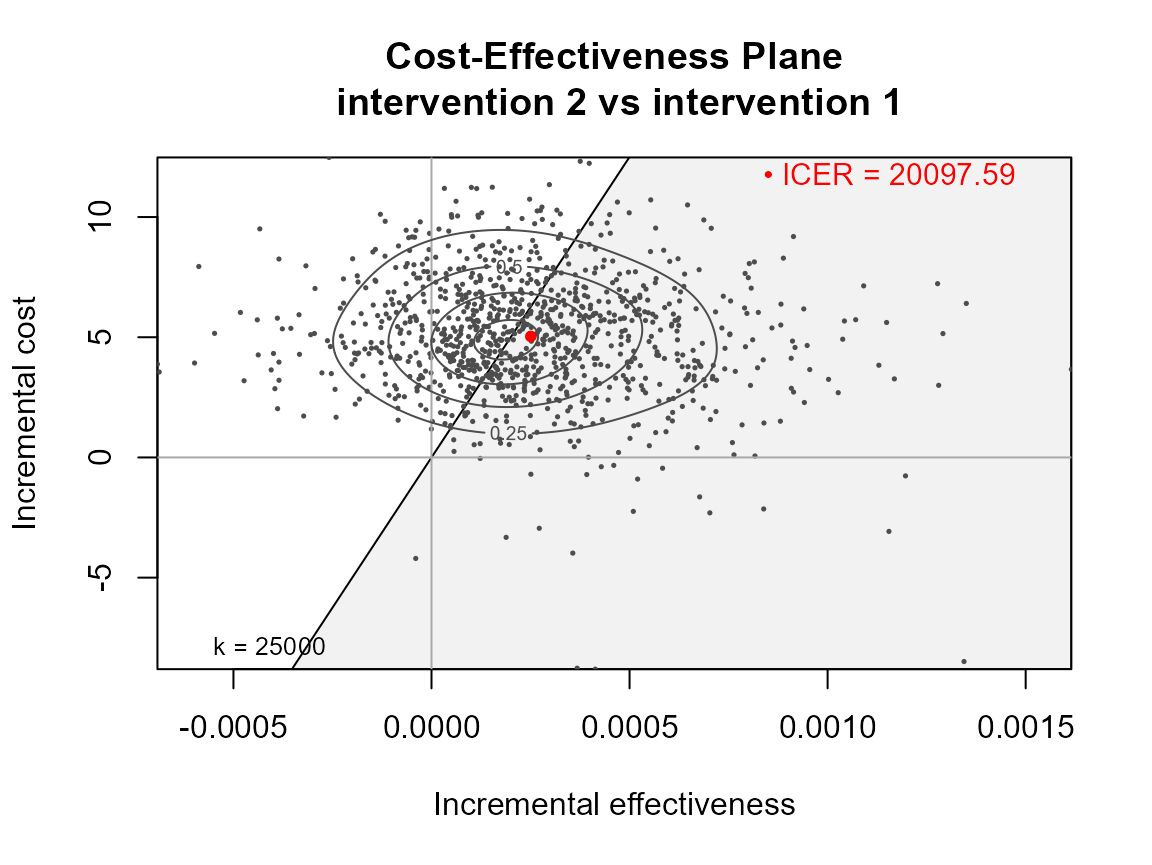contour2(he, graph = "ggplot2")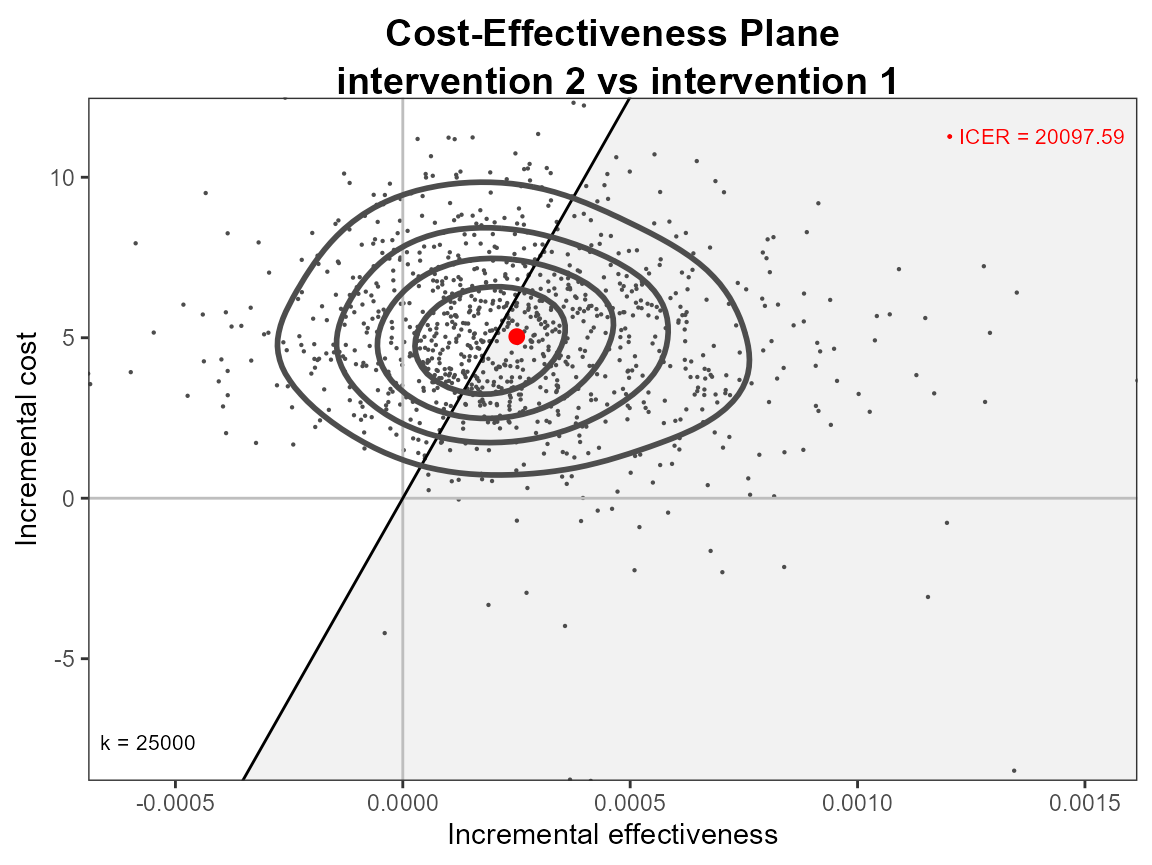# ceac.plot(he, graph = "plotly")

Other plotting arguments can be specified in exactly the same way as above.

contour2(he,
graph = "ggplot2",
title = "my title",
point = list(color = "blue", shape = 10, size = 5),
contour = list(size = 2))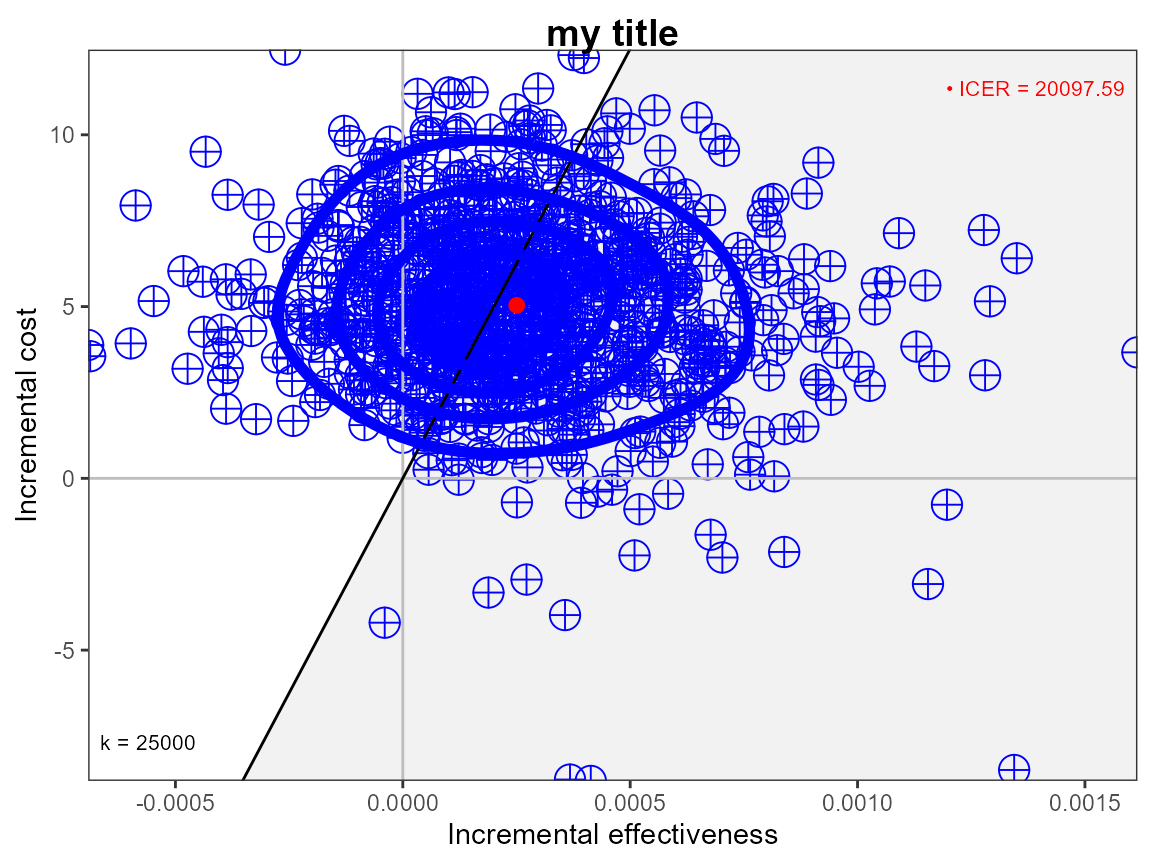contour2(he,
graph = "base",
title = "my title",
point = list(color = "blue", shape = 2, size = 3),
contour = list(size = 4))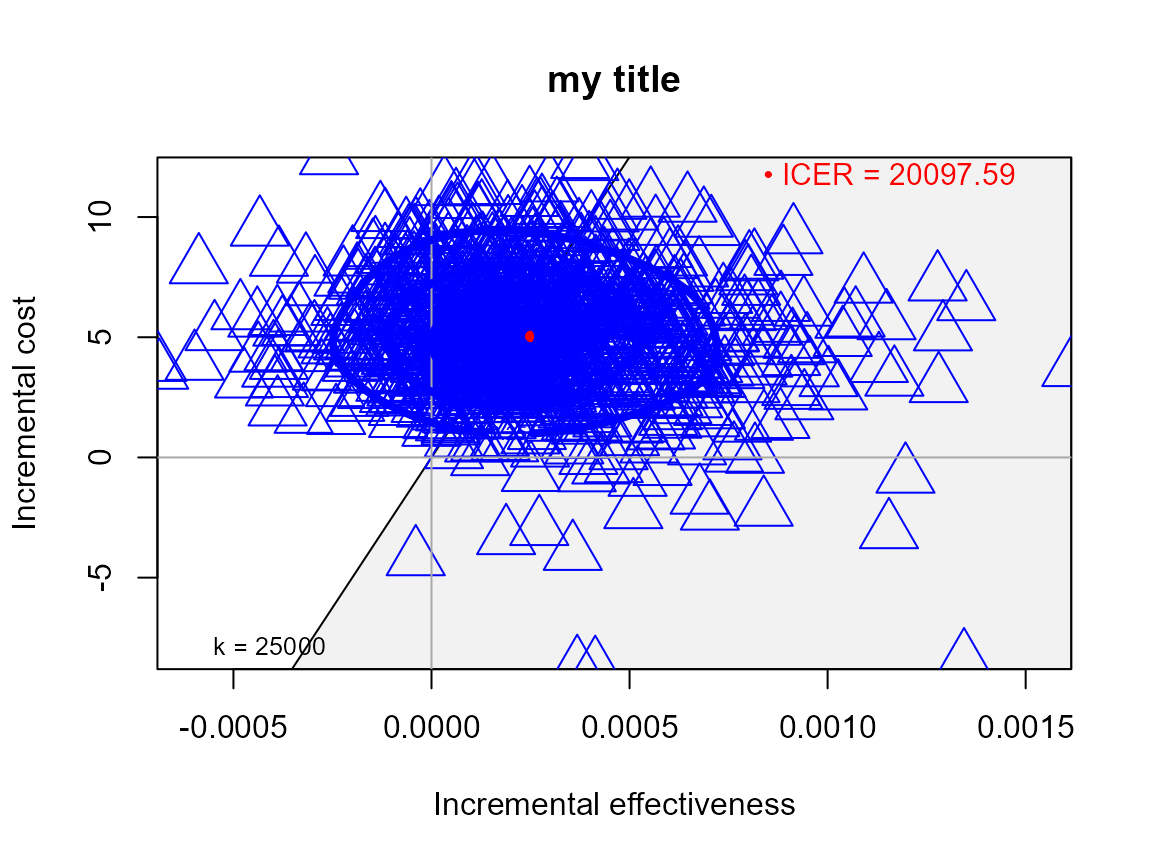## Multiple interventions

This situation is when there are more than two interventions to consider.

#### R code

data("Smoking")
he <- bcea(eff, cost, ref = 4)
# str(he)

Because there are multiple groups then the quadrant annotation is omitted.

contour(he)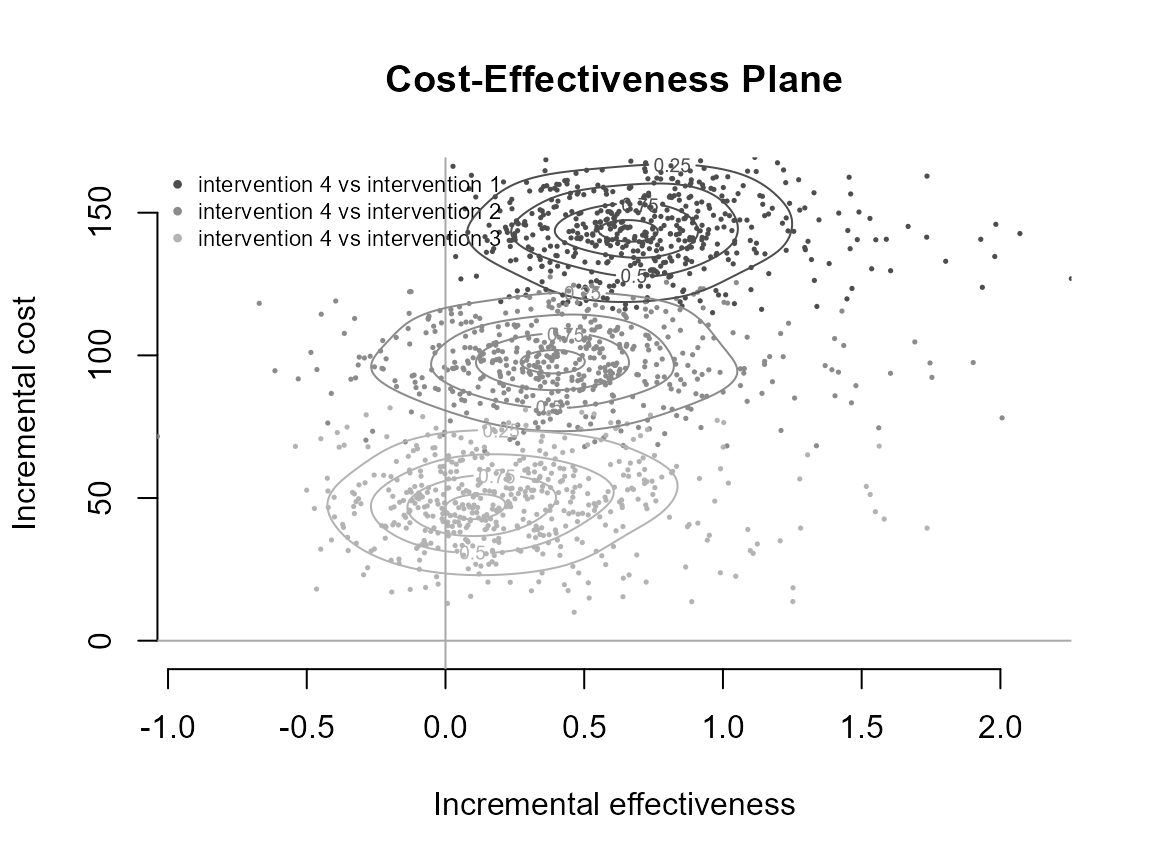contour(he, graph = "ggplot2")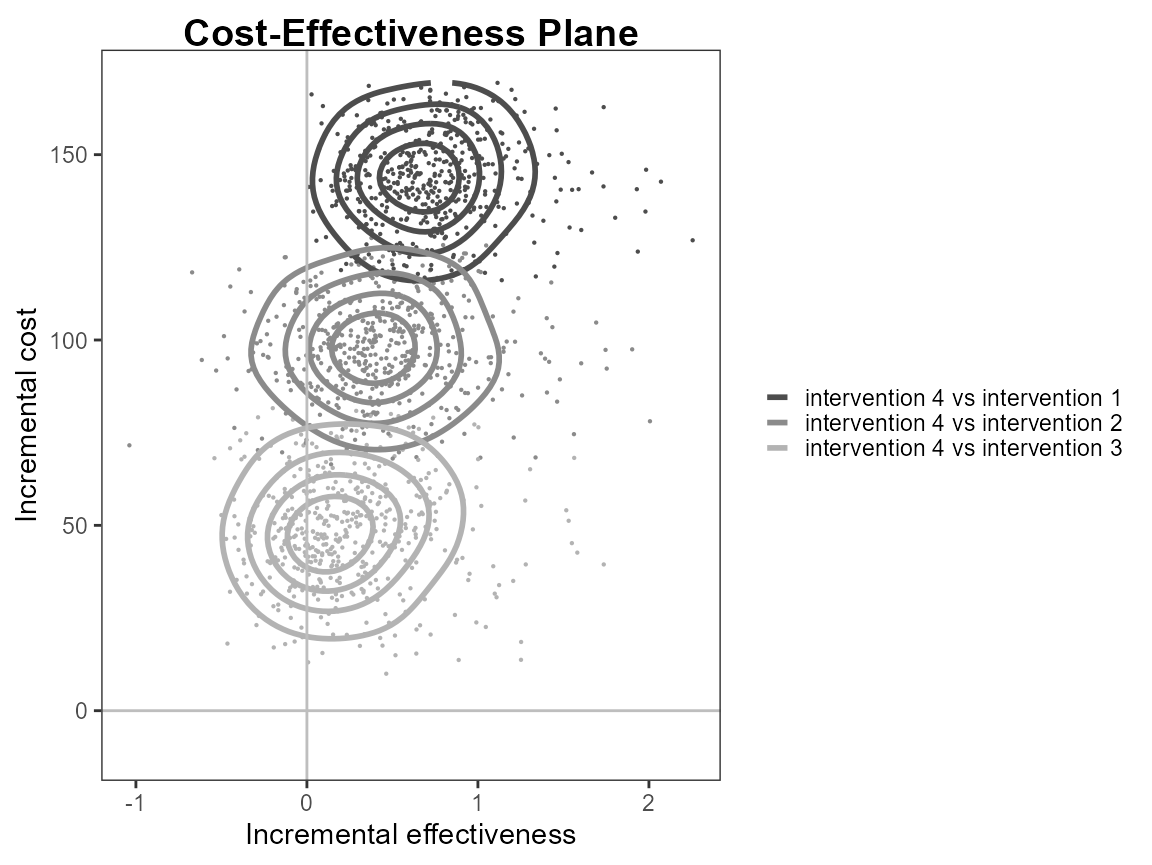The scale argument determines the smoothness of the contours.

contour(he, scale = 0.9)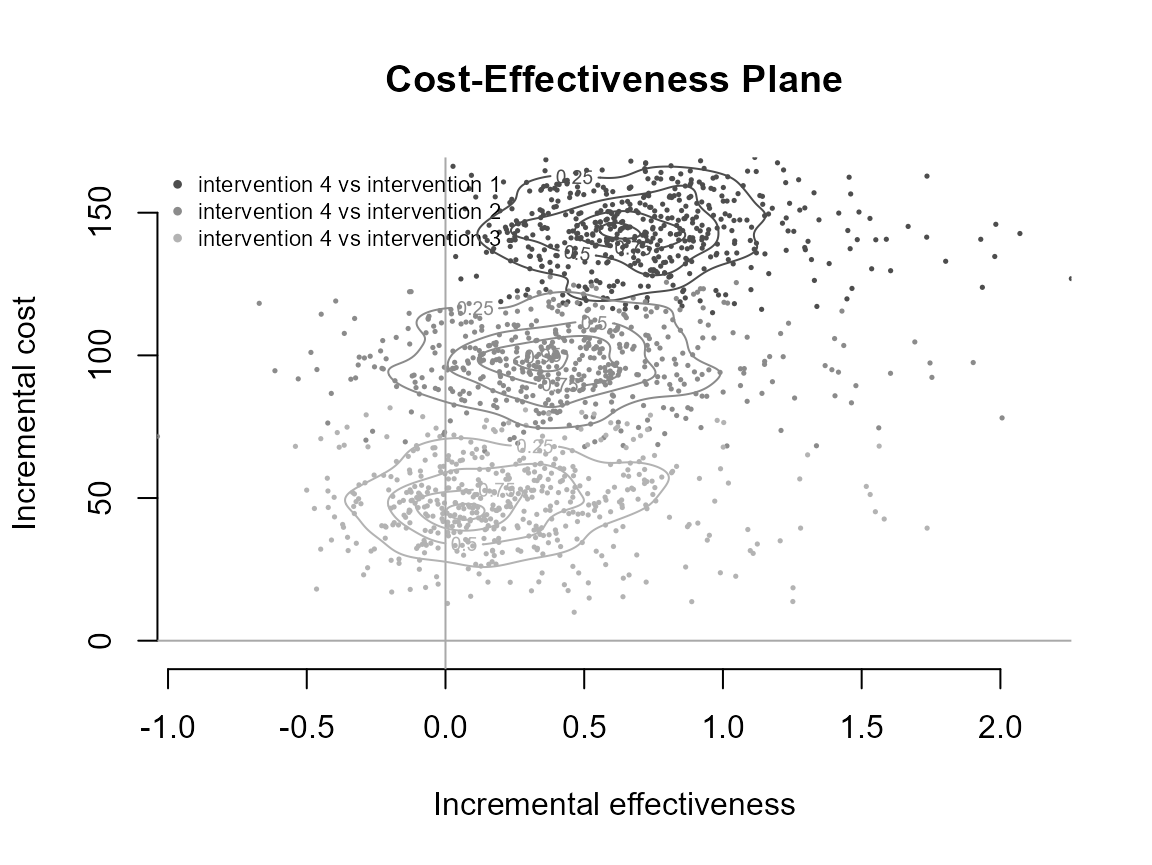contour(he, graph = "ggplot2", scale = 0.9)  ##TODO: what is the equivalent ggplot2 argument?The quantiles or number of levels.

contour(he, nlevels = 10)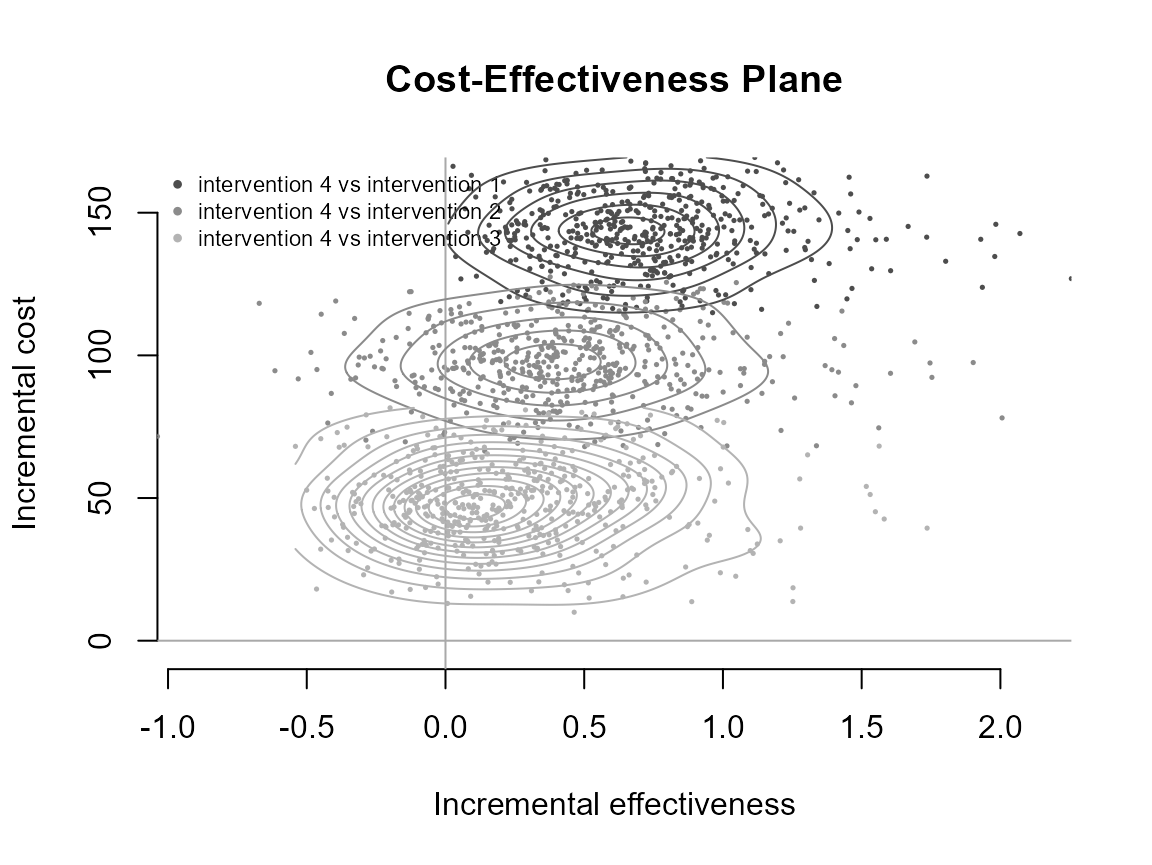contour(he, graph = "ggplot2", contour = list(bins = 10))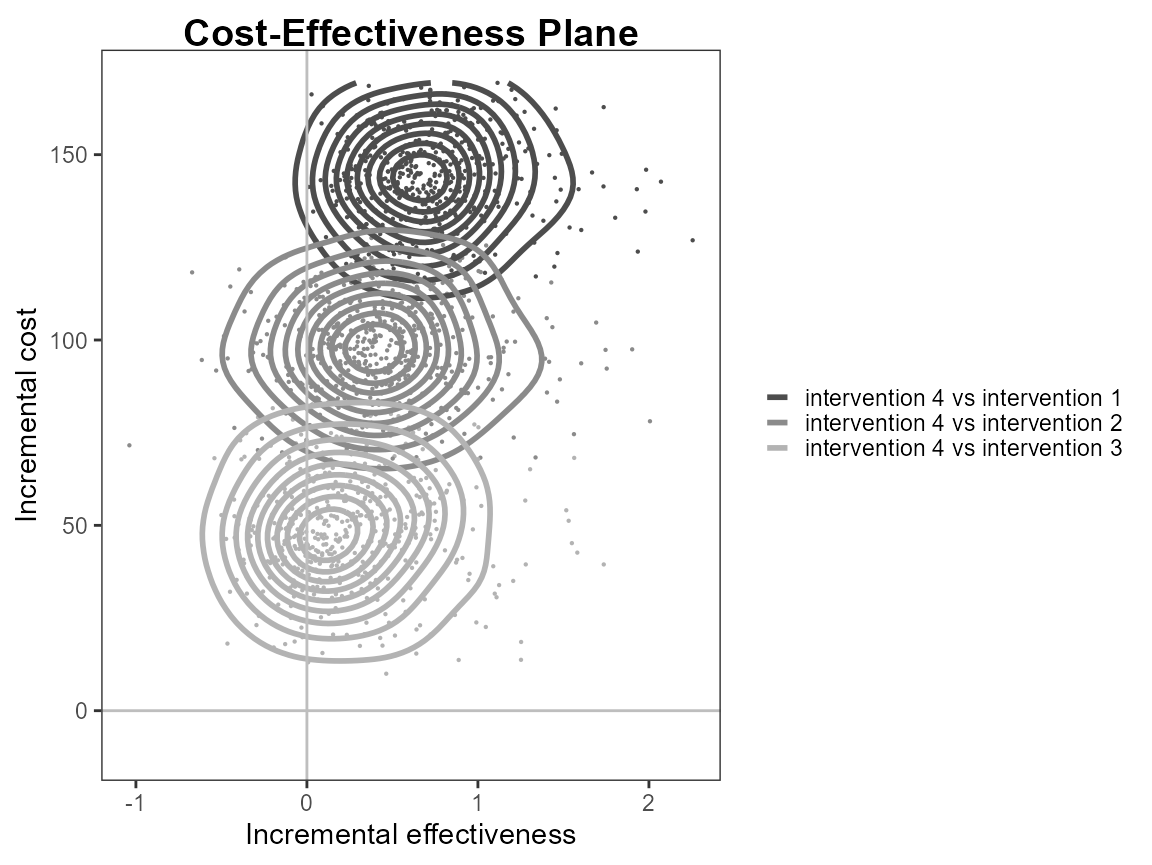contour(he, levels = c(0.2, 0.8))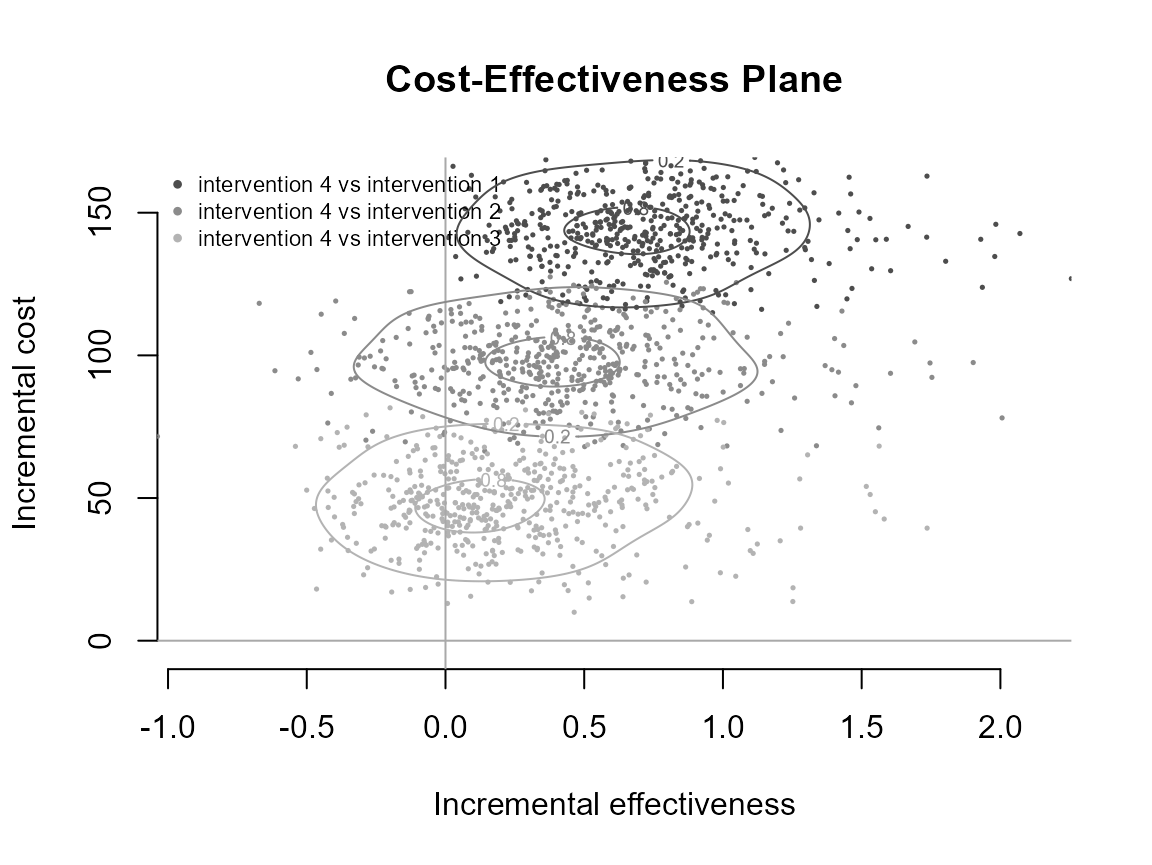contour(he, graph = "ggplot2", contour = list(breaks = c(0.2, 0.8)))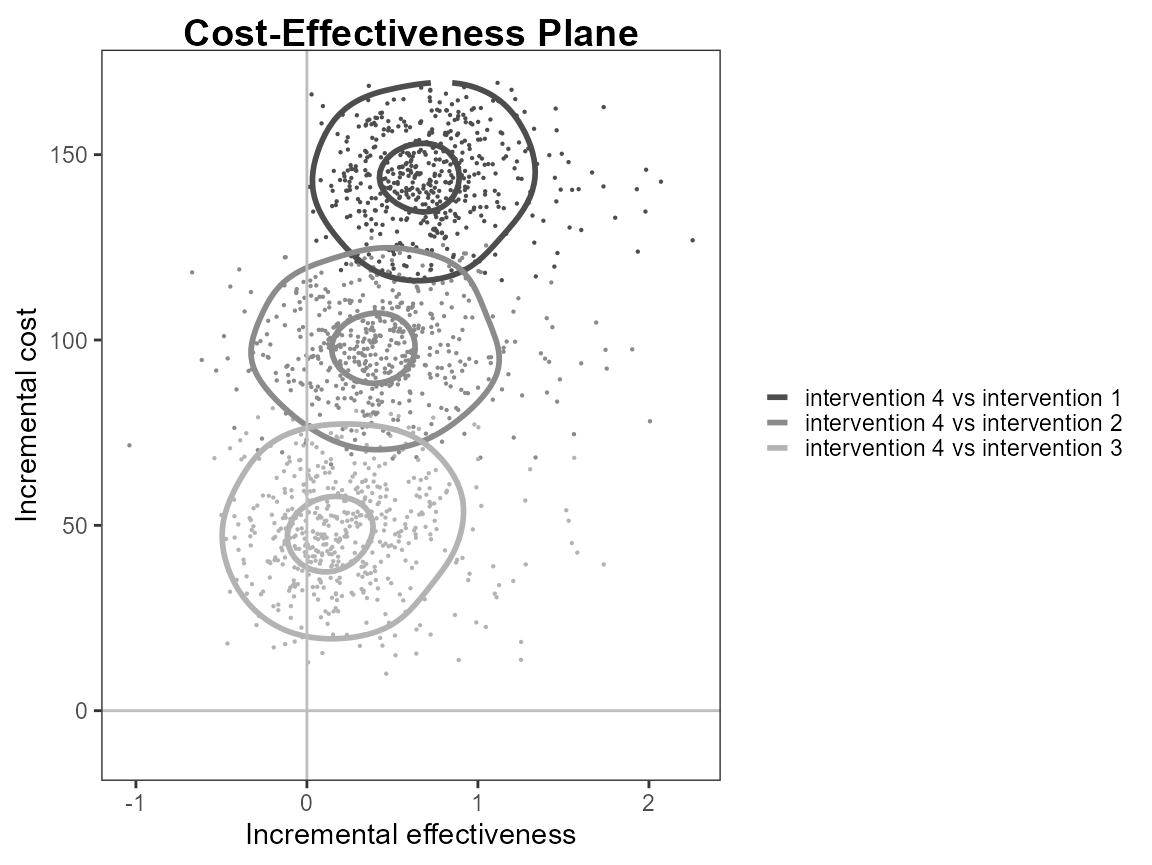contour(he,
graph = "ggplot2",
title = "my title",
line = list(color = "red", size = 1),
point = list(color = c("plum", "tomato", "springgreen"), shape = 3:5, size = 2),
icer = list(color = c("red", "orange", "black"), size = 5),
contour = list(size = 2))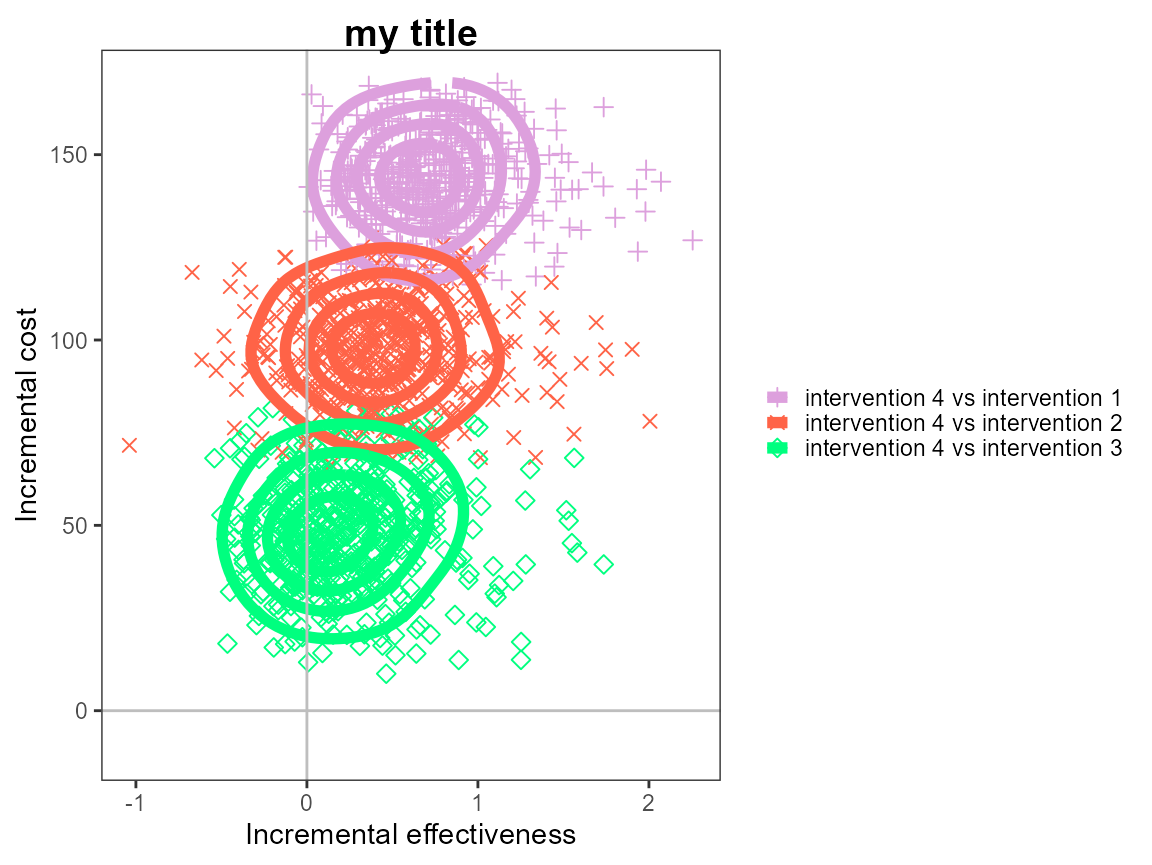contour(he,
graph = "base",
title = "my title",
line = list(color = "red", size = 1),
point = list(color = c("plum", "tomato", "springgreen"), shape = 3:5, size = 2),
icer = list(color = c("red", "orange", "black"), size = 5),
contour = list(size = 4))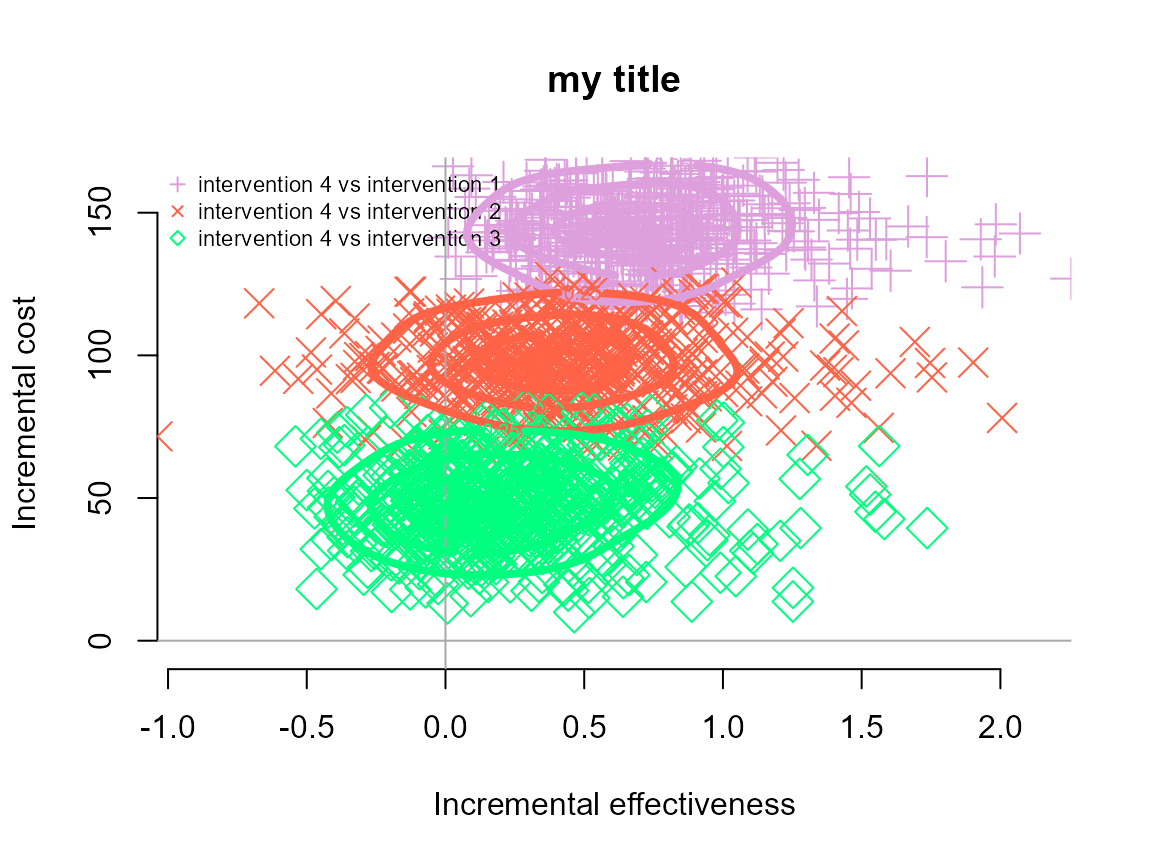Again, this applies to the contour2() version of contour plot too.

contour2(he, wtp = 250)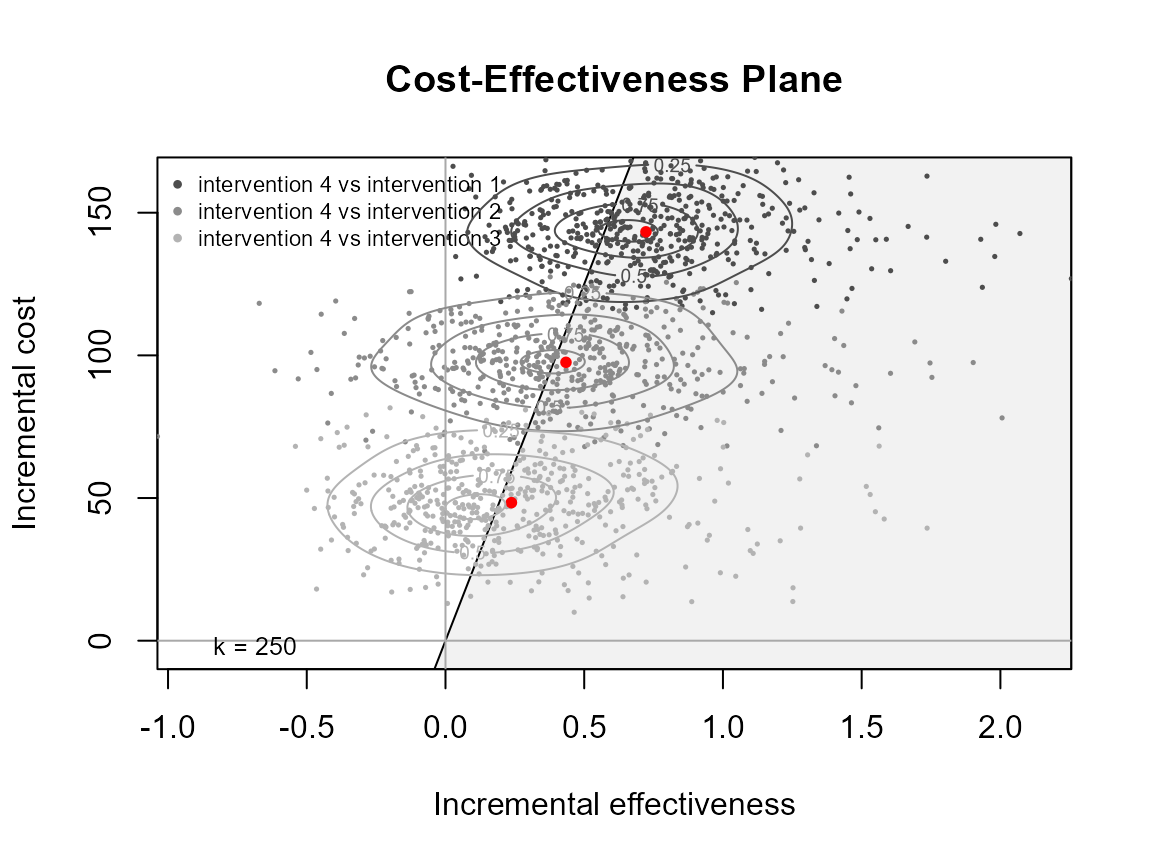contour2(he, wtp = 250, graph = "ggplot2")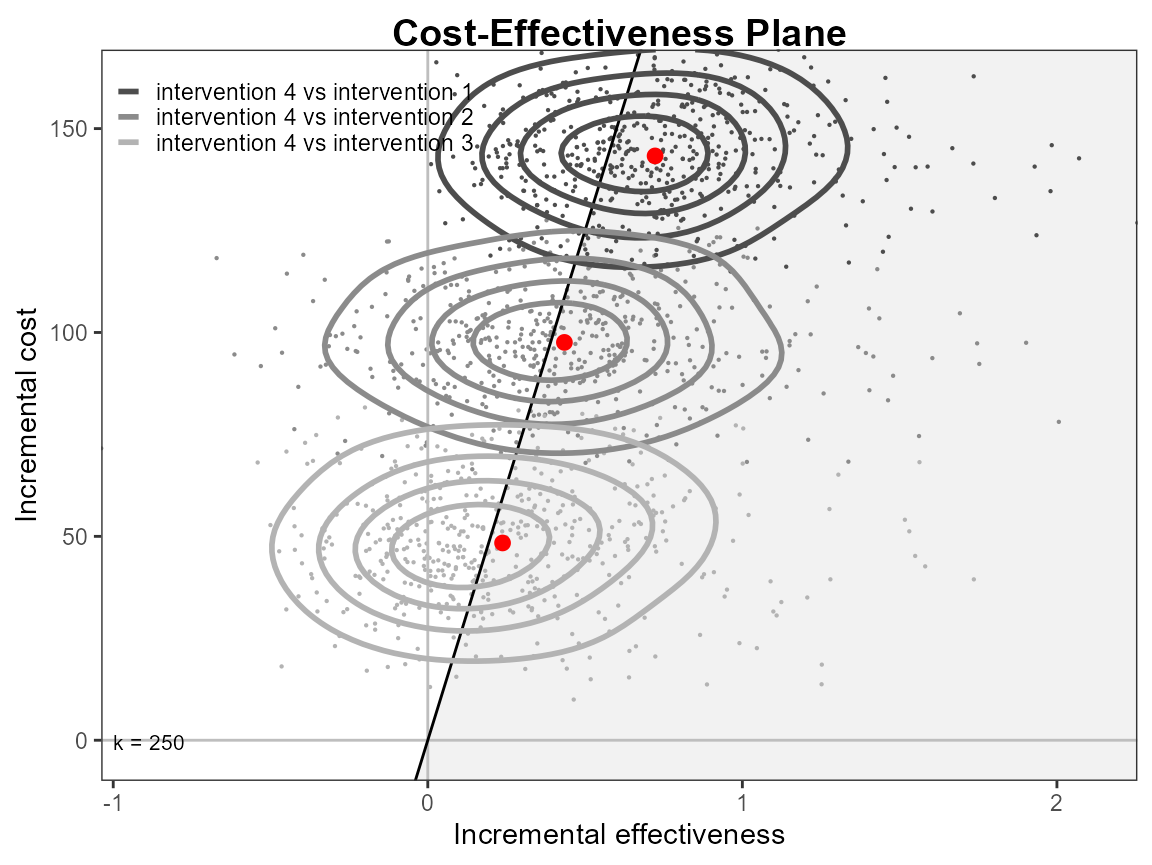The styling of the plot for multiple comparisons can specifically change the colour and point type for each comparison.

contour2(he, wtp = 250,
graph = "ggplot2",
title = "my title",
line = list(color = "red", size = 1),
point = list(color = c("plum", "tomato", "springgreen"), shape = 3:5, size = 2),
icer = list(color = c("red", "orange", "black"), size = 5),
contour = list(size = 2))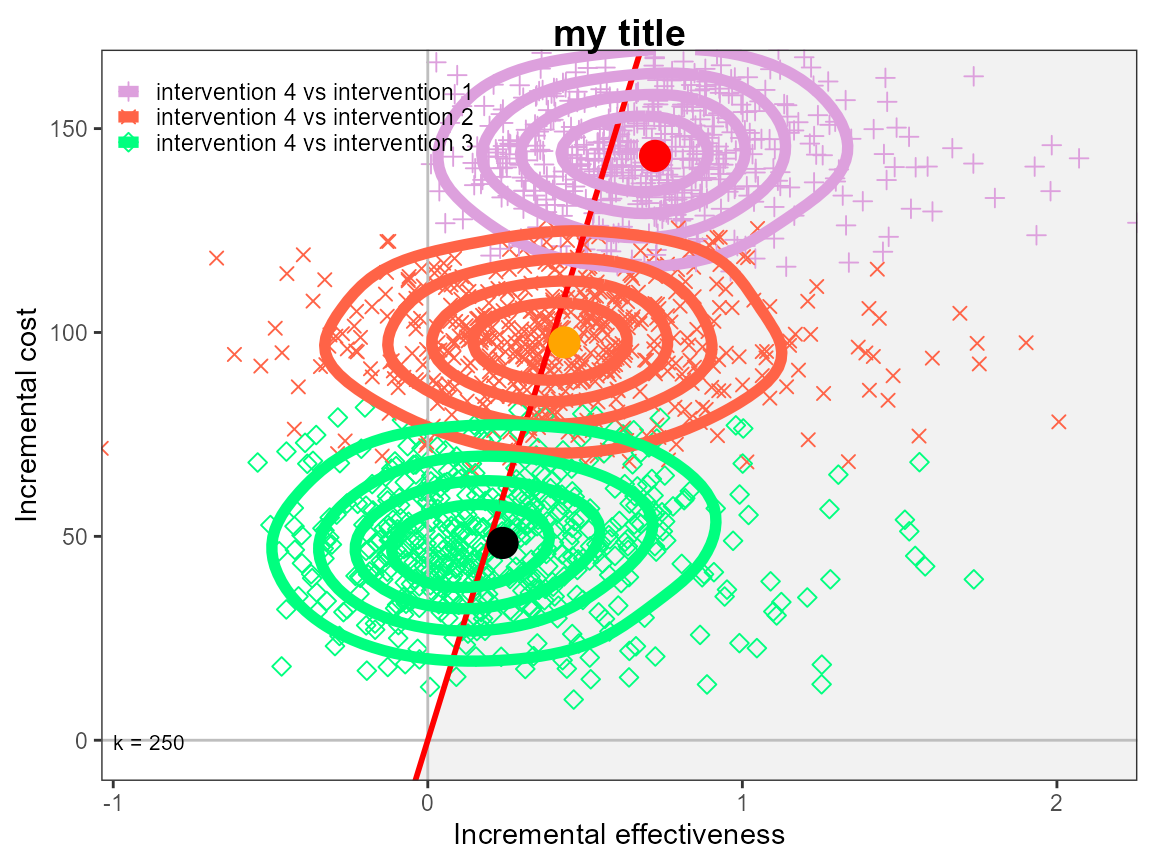contour2(he, wtp = 250,
graph = "base",
title = "my title",
line = list(color = "red", size = 1),
point = list(color = c("plum", "tomato", "springgreen"), shape = 3:5, size = 2),
icer = list(color = c("red", "orange", "black"), size = 5),
contour = list(size = 4))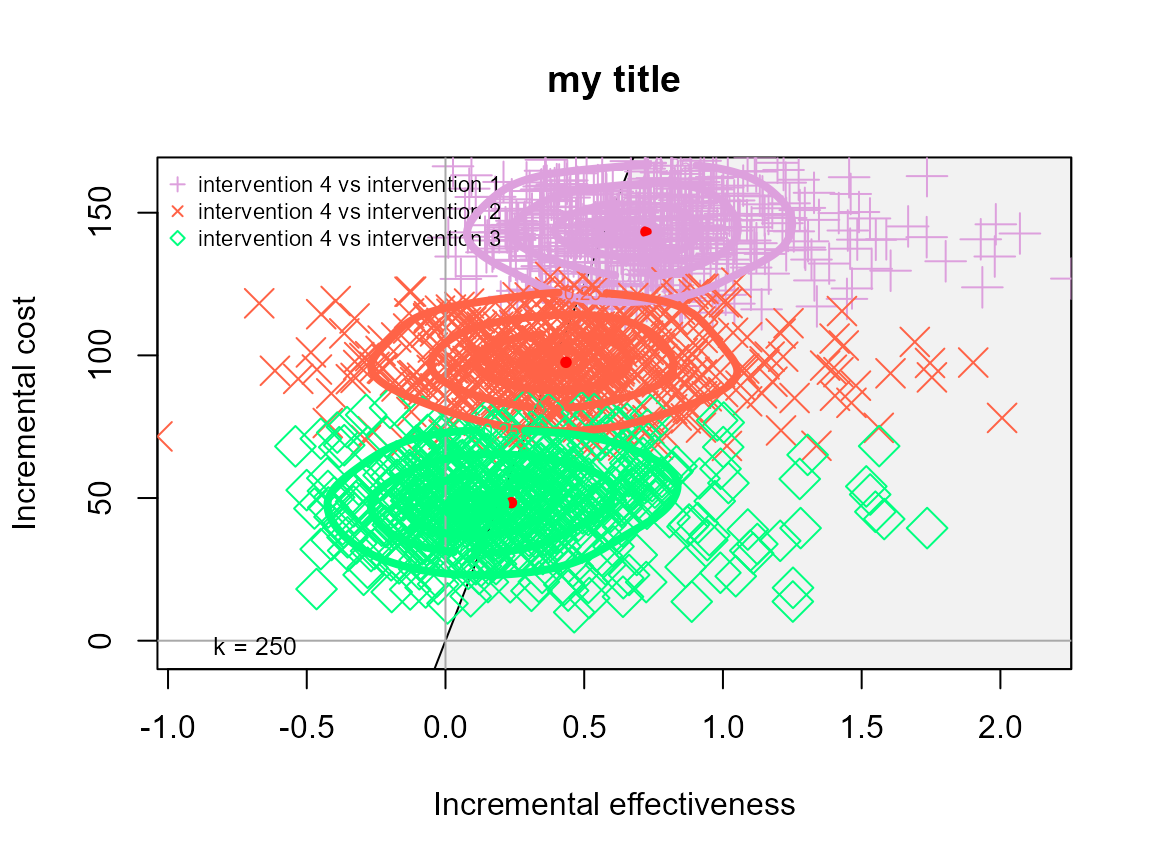Reposition legend.

contour(he, pos = FALSE)    # bottom right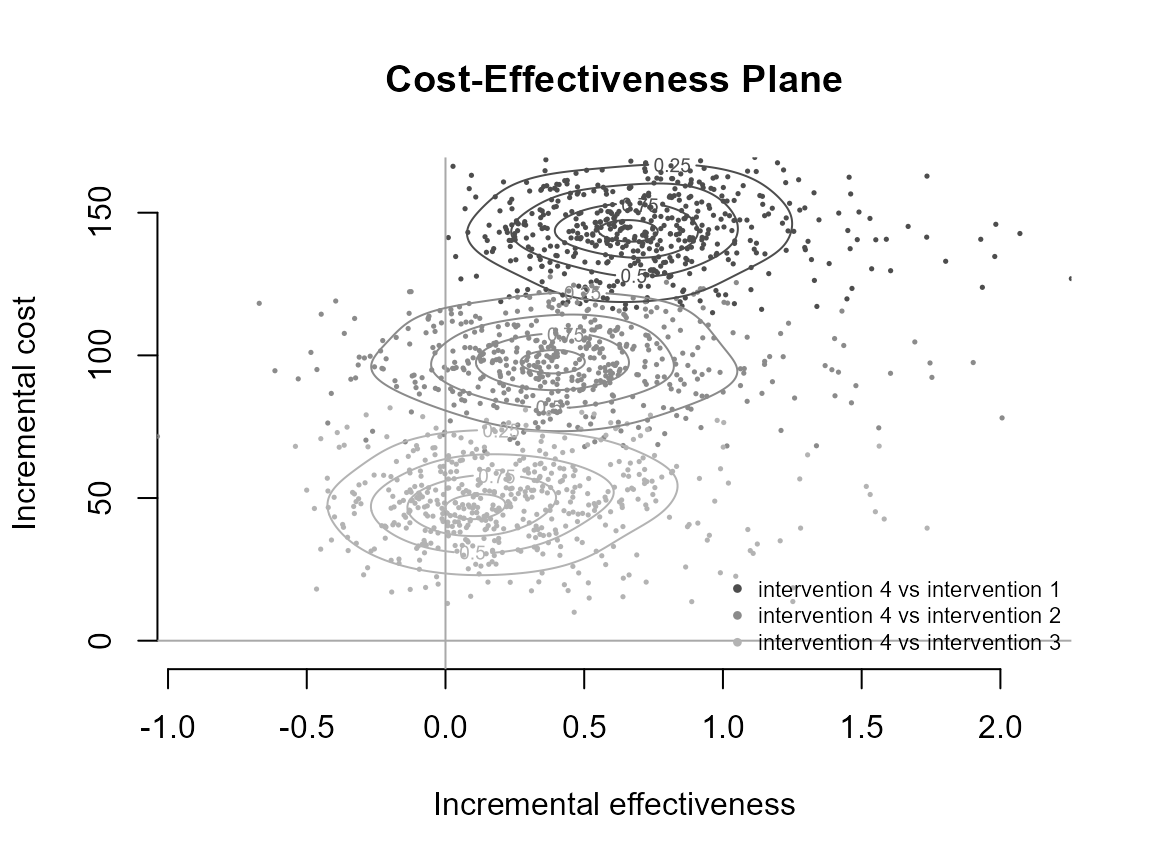contour(he, pos = c(0, 0))contour(he, pos = c(0, 1))contour(he, pos = c(1, 0))contour(he, pos = c(1, 1))